Author: Oscar Cronquist Article last updated on March 25, 2020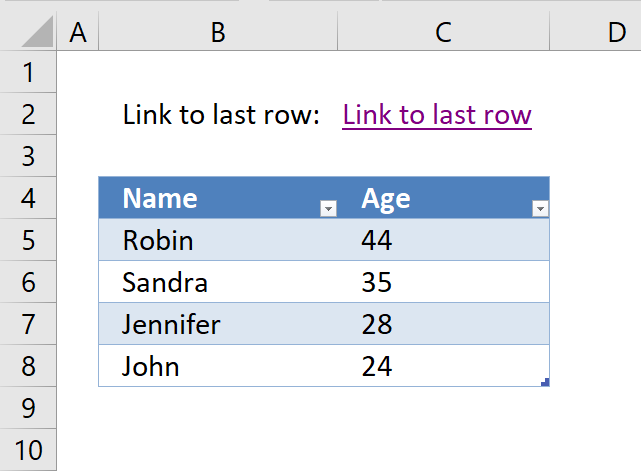This article will demonstrate how to create a hyperlink that takes you to the first empty cell below data in a column. The way it works is that the Excel user press with left mouse button ons on the hyperlink and Excel instantly takes you to the first empty cell in a column.

The image above shows the formula in cell C2, it creates a hyperlink pointing to the first empty cell below the data set. This means that if more values are added to the data set the formula adapts and changes the destination cell making it dynamic without any user interaction.

This method can make the worksheet more user-friendly and easier to navigate, there is no VBA code or User Defined Functions in this workbook. The HYPERLINK function is a really versatile and interesting function that can perform many useful actions.

Formula in cell C2: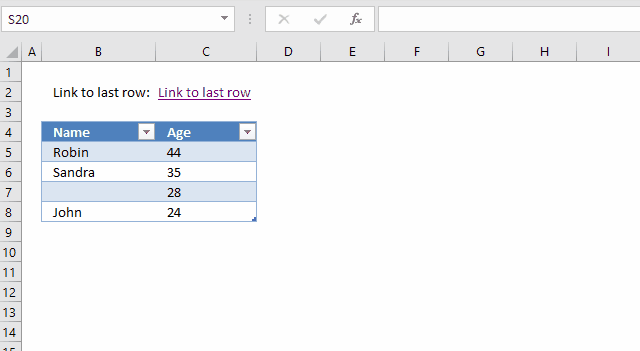The hyperlink takes you to the first empty cell below the data set even if you have one or many blanks in the data set. The animated image above demonstrates this, the data set is not large in this example but you get the idea.

### Explaining the formula in cell C2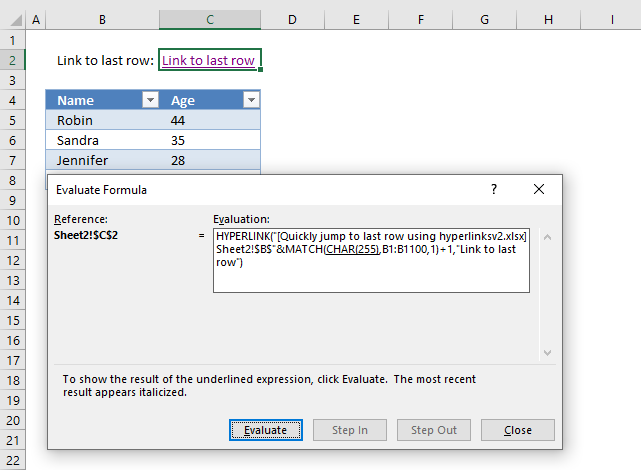I recommend using the "Evaluate Formula" tool which is a feature built-in to Excel. It allows you to see the formula calculation step by step.

Go to tab "Formulas" on the ribbon, press with left mouse button on the "Evaluate Formula" button and a dialog box opens, see image above.

The step that is about to be calculated is underlined, when you press with left mouse button on the "Evaluate" button the underlined expression is calculated. The calculated step is in italic.

Use the scroll arrows if the formula is larger than the window, this lets you see the entire formula. I wish it was possible to make that evaluation window larger though.

Press with left mouse button on the "Evaluate" button to move to the next step in the calculation. Continue press with left mouse button oning the "Evaluate" button to see all calculation steps in the formula. Press with left mouse button on "Close" button to dismiss the dialog box.

#### Step 1 - Find the last nonempty cell in cell range B1:B100

The CHAR function converts a number to the corresponding character based on your computer's character set. The numbers can be from 1 to 255 and they represent a character.

MATCH(CHAR(255),B1:B100,1)

becomes

MATCH(ÿ,B1:B100,1)

The MATCH function returns the relative position of a given value in a cell range. The third argument lets you choose how the function matches the value. Number 1 let you find the smallest value that is greater than or equal to the lookup value.

MATCH(lookup_value, lookup_array, [match_type])

We are looking for the last character in your computer's character set and it will most likely not be found. This will match the last value in your lookup_array even if it is not sorted in ascending order.

If you know that your lookup_array contains this value then I recommend using multiple values concatenated like this CHAR(255)&CHAR(255).

MATCH(ÿ,B1:B100,1)

and returns 8 which is the last value in cell range B1:B100.

If your data set has more than 100 rows use a larger cell range. For example B1:B1000 or the entire column B:B, however, the formula will probably run slower.

#### Step 2 - Join file name, sheet and cell address

The HYPERLINK function requires the file name, worksheet name and a cell reference to work properly. Step 1 above calculated the row number of the last non-empty cell.

We need to add the file name, worksheet name and column letter, then concatenate this string with the row number. The workbook name has a beginning and ending bracket characters and the worksheet name ends with a ! (exclamation mark).

We need to reference the first empty cell below the last non-empty value, in order to do that we add 1 to the calculated row number.

becomes

and returns

#### Step 3 - Create the hyperlink

The HYPERLINK function lets you build a hyperlink in your worksheet using a formula. A formula can be made dynamic so if your data set changes the formula changes as well without any user interaction.

The HYPERLINK function has two arguments, the first one is the link location. We built the link location in step 1 and 2. The friendly name is optional, I chose the word "Hyperlink". However, I recommend you use something more descriptive.

becomes

### Tip 1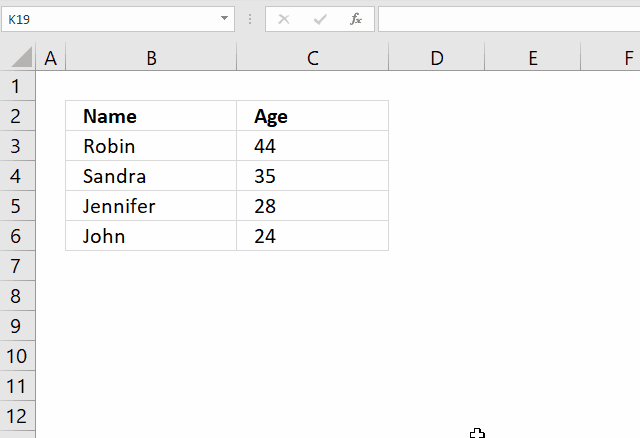You can also use this keyboard shortcut to find the last non-empty cell:

1. Select any cell in the column.
2. Ctrl + Arrow key down.

However, if there are many blanks in your column you must repeatedly press the short cut keys to reach the last one.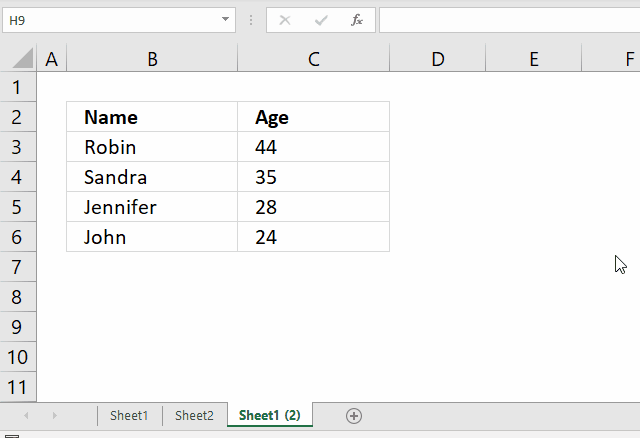Go to a column that has no values, press CTRL + arrow key down. This takes you to the last cell in that column. Use the arrow keys or your mouse to select any cell in the column you want to find the last value in.

Now press CTRL + arrow key up and Excel takes you to the last non-empty cell in that column.

### Get the Excel file